## Permutation - Neon instructions

Neon provides several different kinds of permute instruction to perform different operations:

## Move instructions

The move instructions copy a sequence of bits into a register. This bit sequence can come either from another register or from a compile-time constant.

The `MOV` instruction has several variants, as shown in the following table:

Instruction Description
`MOV X0, #2` Set X0 to 2.
`MOV X0, X1` Set X0 to the value of X1.
`MOV X0, V3.S`

Set X0 to the value of the second single word (bits 32-63) in V0.

This instruction is an alias of `UMOV`.

`MOV V0, V2.H`

Set every halfword (16 bit) lane in V0, to the value in the third halfword lane of V2.

This instruction is an alias of `DUP`.

`MOV V2.S, S0 `

Set the third single-word lane in V2, to the value of S0.

This instruction is an alias of `INS`.

`MOV s0, v2.S`

Set S0, to the value in the third single-word lane of V2.

This instruction is an alias of `INS`.

The following move instructions specify a sign extension:

Instruction Description
`UMOV X0, V3.S` Set X0 to the zero-extended value of the second single in V3.
`SMOV X0, V3.S` Set X0 to the sign-extended value of the second single in V3.

The following move instructions operate on floating-point values:

The following move instructions specify a sign extension:

Instruction Description
`FMOV S0, #1.0` Set S0, the lowest 32 bits of V0, to the floating-point value 1.0.
`FMOV V0.8H, #2.0` Set all eight halfword (16-bit) lanes in V0 to the floating-point value 2.0.
`FMOV D1, D4` Set D1 to the value of D4.

All these move instructions have the following in common:

• The instructions copy a single fixed sequence of bits into one or more lanes in a destination register.
• The instructions do not perform any floating-point type conversion.

If you need to move more than one value, see the other instructions below. Floating point conversions are beyond the scope of this guide.

## Reverse instructions

The reverse instructions break a vector into ordered containers. The ordering of these containers is preserved. These containers are then split into ordered subcontainers. Within each container, the ordering of subcontainers is reversed. The newly ordered elements are then copied into the destination register.

For example, consider the following instruction:

`REV16 v0.16B, v1.16B`

This instruction splits the 128-bit V1 register into eight 16-bit halfword containers. Each of these halfword containers is then split into a pair of one-byte subcontainers. Each pair of subcontainers is then reversed, as shown in the following diagram: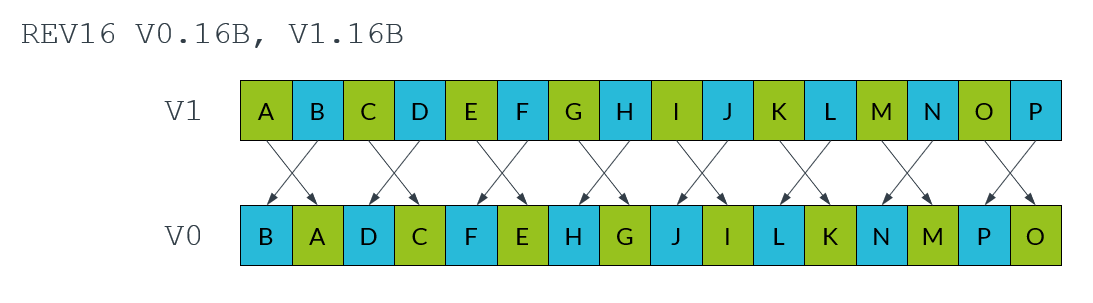There are several reverse instructions to handle different sizes of containers and subcontainers, as shown in the following tables and diagrams:

Instruction Number of containers Size of containers Number of subcontainers in each container Size of subcontainers
`REV16 v0.16B, v1.16B` 8 16-bit 2 8-bit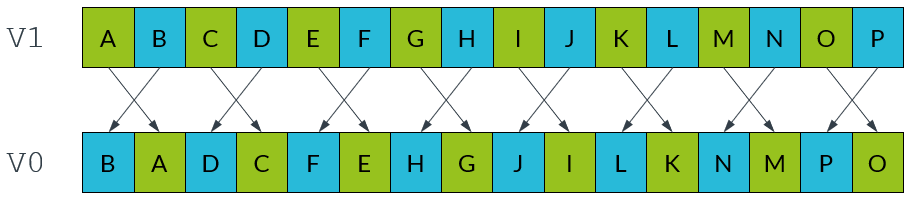Instruction Number of containers Size of containers Number of subcontainers in each container Size of subcontainers
`REV32 v0.16B, v1.16B ` 4 32-bit 4 8-bit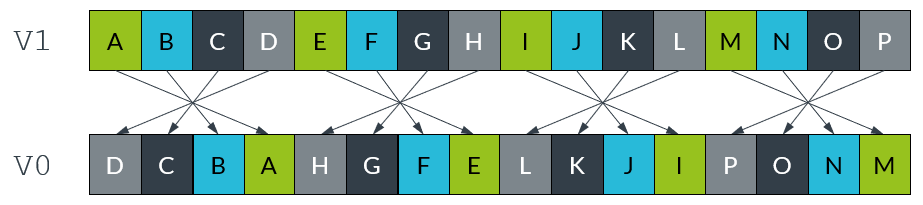Instruction Number of containers Size of containers Number of subcontainers in each container Size of subcontainers
`REV32 v0.8H, v1.8H` 4 32-bit 2 16-bit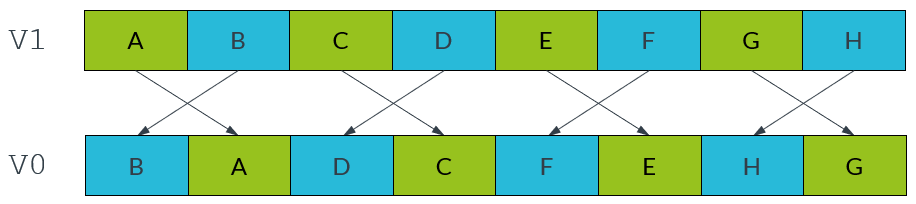Instruction Number of containers Size of containers Number of subcontainers in each container Size of subcontainers
`REV64 v0.16B, v1.16B` 2 64-bit 8 8-bit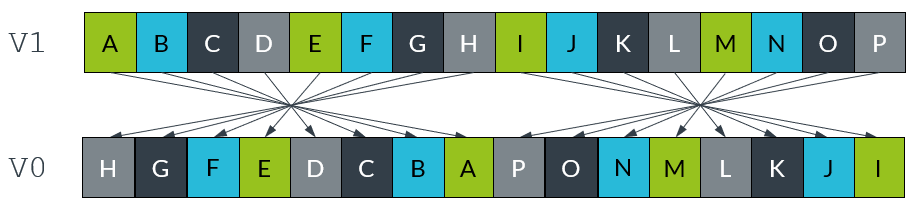Instruction Number of containers Size of containers Number of subcontainers in each container Size of subcontainers
`REV64 v0.8H, v1.8H` 2 64-bit 2 16-bit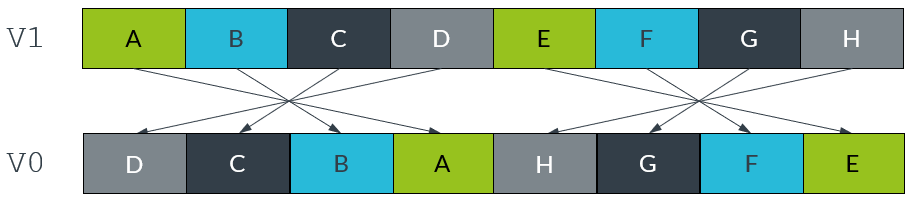Instruction Number of containers Size of containers Number of subcontainers in each container Size of subcontainers
`REV64 v0.4S, v1.4S` 2 64-bit 2 32-bit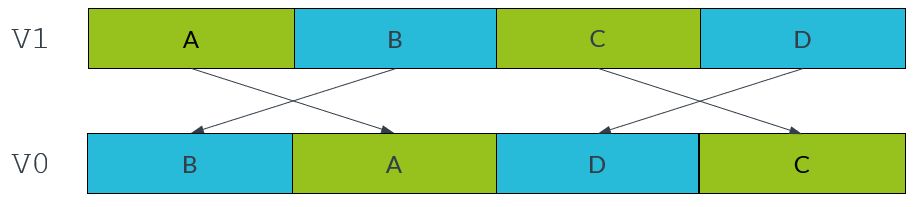## Extraction instructions

The extract instruction, `EXT`, creates a new vector by extracting consecutive lanes from two different source vectors. An index number, n, specifies the lowest lane from the first source vector to include in the destination vector. This instruction lets you create a new vector that contains elements that straddle a pair of existing vectors.

The `EXT` instruction constructs the new vector by doing the following:

1. From the first source vector, copy the lower n lanes to the highest lanes in the destination vector.
2. From the second source vector, ignore the lower n lanes and copy the remaining lanes to the lowermost lanes in the destination vector.

For example, the following instruction uses an index with value 3:

`EXT v0.16B, v1.16B, v2.16B, #3`

This instruction extracts lanes as follows:

1. Copy the lowest 3 bytes from V1 into the highest 3 bytes of V0.
2. Copy the highest 13 bytes of V2 into the lowest 13 bytes of V1.

The following diagram illustrates the extraction process: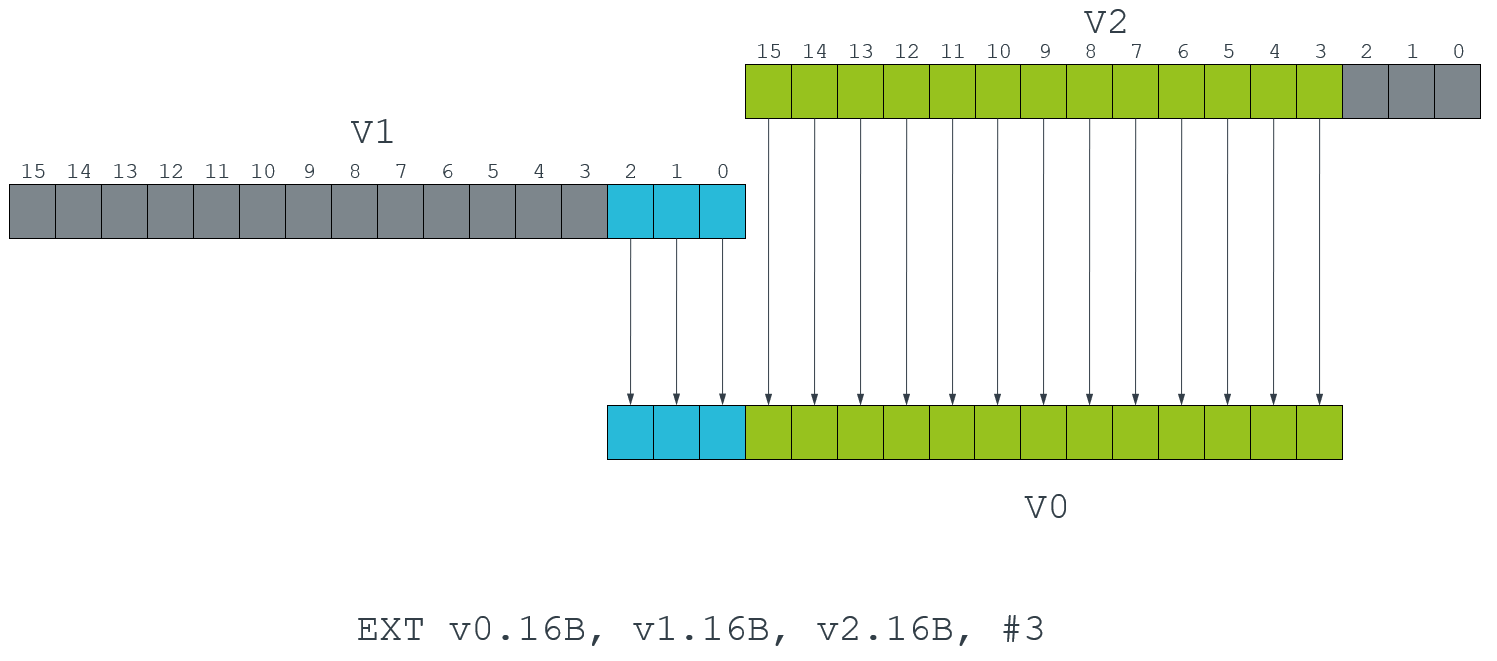The other extraction instructions are less general. They copy all the values from a source register, then place them into smaller lanes in the destination, as follows:

• `XTN` Extract and narrow

Reads each vector element from the source register, narrows each value to half the original width, and writes the resulting vector to the lower half of the destination register. The upper half of the destination register is cleared.

The following diagram shows the operation of the `XTN` instruction: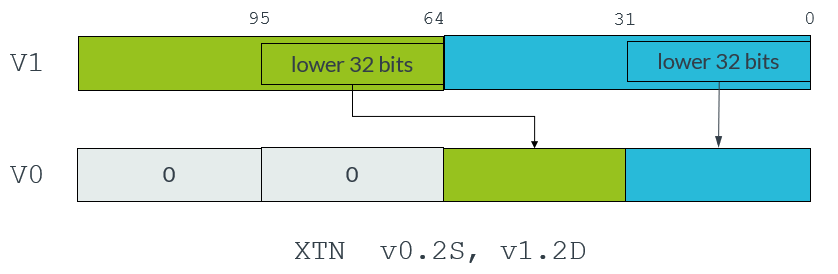• `XTN2` Extract and narrow into upper halves

Reads each vector element from the source register, narrows each value to half the original width, and writes the resulting vector to the upper half of the destination register. The other bits of the destination register are not affected.

The following diagram shows the operation of the `XTN2` instruction: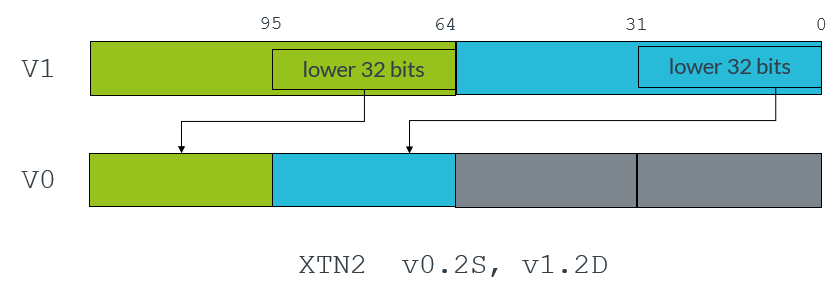With both the `XTN` and `XTN2` instructions, the destination vector elements are half as long as the source vector elements.

Neon provides several variants of the extraction instructions for different combinations of sign and overflow behavior. The following table shows these extraction instruction variants:

Table 1‑1

 Instruction Description `SQXTN` Signed saturating extract and narrow. All values are signed integer values. Large values saturate to the maximum positive or negative integer value. `SQXTN2` Signed saturating extract and narrow into upper halves. All values are signed integer values. Large values saturate to the maximum positive or negative integer value. `SQXTUN` Signed saturating extract and unsigned narrow. Source values are signed, destination values are unsigned. Large values saturate to the maximum positive integer value or zero. Other values are zero extended. `SQXTUN2` Signed saturating extract and unsigned narrow into upper halves. Source values are signed, destination values are unsigned. Large values saturate to the maximum positive integer value or zero. Other values are zero extended. `UQXTN` Unsigned saturating extract and narrow. All values are unsigned integer values. Large values saturate to the maximum positive integer value or zero. Other values are zero extended. `UQXTN2` Unsigned saturating extract and narrow into upper halves. All values are unsigned integer values. Large values saturate to the maximum positive integer value or zero. Other values are zero extended.

## Transpose instructions

The transpose instructions interleave elements from two source vectors. Neon provides two transpose instructions: `TRN1` and `TRN2`.

`TRN1` interleaves the odd-numbered lanes from the two source vectors, while `TRN2` extracts the even-numbered lanes. The following diagram shows this process: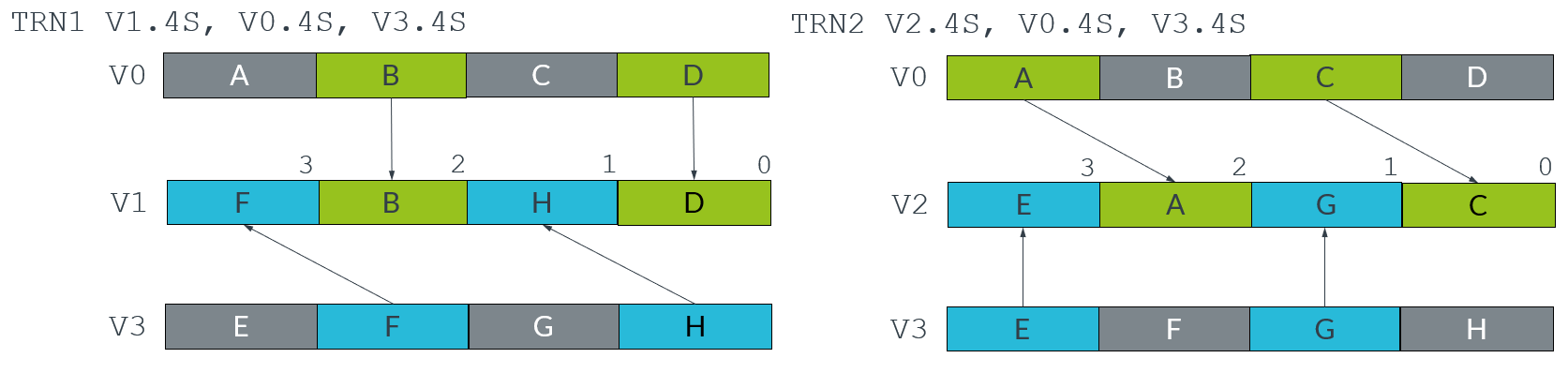In mathematics, the transpose of a matrix is an operation that switches the rows and columns. For example, the following diagram shows the transpose of a 2x2 matrix: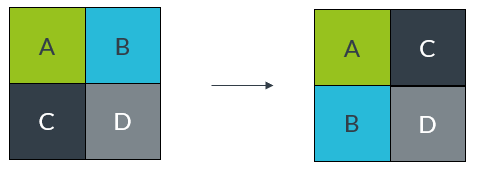We can use the Neon transpose instructions to transpose matrices.

For example, consider the following two matrices: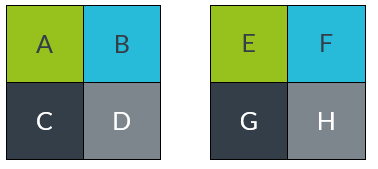We can store these matrices across two Neon registers, with the top row in V0 and the bottom row in V1, as shown in the following diagram: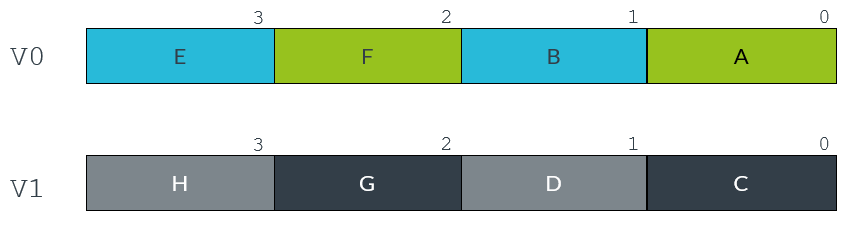The following instructions transpose this matrix into the destination registers V2 and V3:

```TRN1 v2.4s, v0.4S, v1.4S
TRN2 v3.4s, v0.4S, v1.4S```

The following diagram illustrates this process: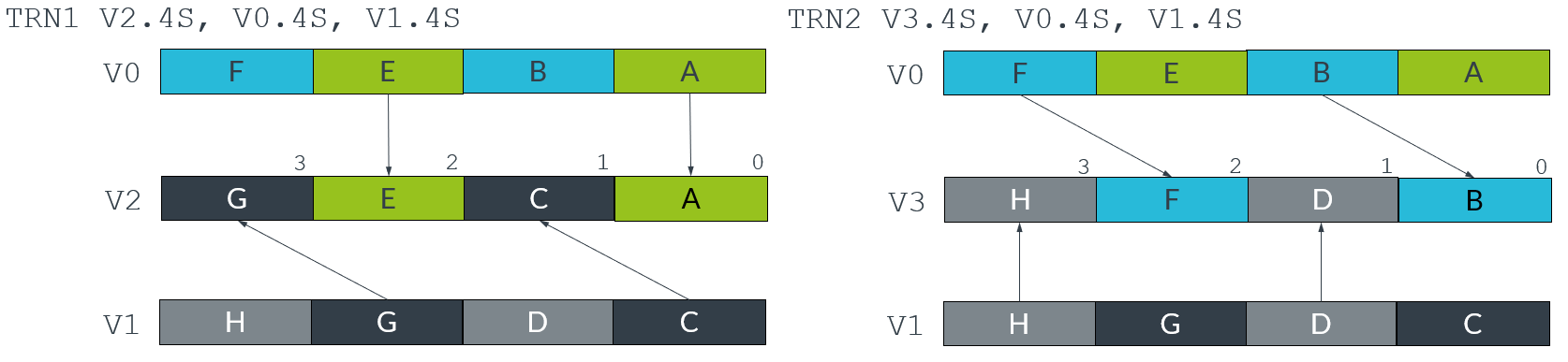The following diagram shows the transposed matrices: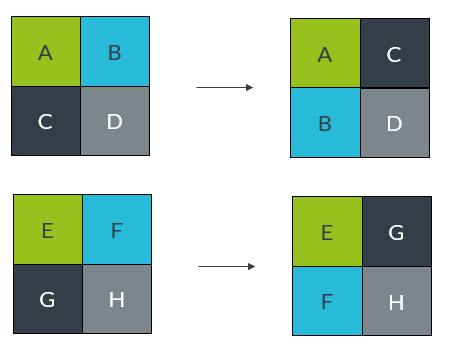## Interleave instructions

Like the transpose instructions, the zip instructions use interleaving to form vectors. `ZIP1` takes the lower halves of two source vectors, and fills a destination vector by interleaving the elements in those two lower halves. `ZIP2` does the same thing with the upper halves of the source vectors.

For example, the following instructions create an interleaved vector that is stored across two registers, V1 and V2:

```ZIP1 V2.16B, V4.16B, V3.16B
ZIP2 V1.16B, V4.16B, V3.16B```

This result vector is formed by alternating elements from the two source registers, V1 and V2. The `ZIP1` instruction creates the lower half of the result vector in V2, and the `ZIP2` instruction creates the upper half in V1. The following diagram shows this process: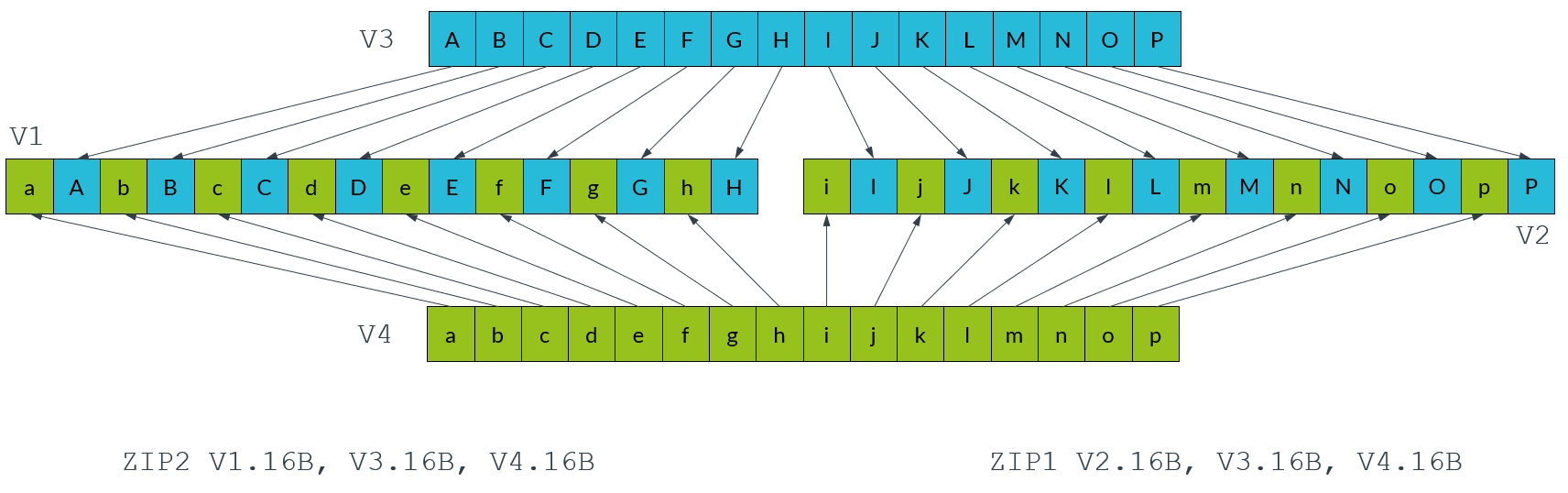The `UZIP1` and `UZIP2` instructions perform the reverse operation, deinterleaving alternate elements into two separate vectors.

## Table lookup instructions

All the permute instructions that we have described have one thing in common: the pattern of the permutation is fixed. To perform arbitrary permutations, Neon provides the table lookup instructions `TBL` and `TBX`.

The `TBL` and `TBX` instructions take two inputs:

• An index input, consisting of one vector register containing a series of lookup values
• A lookup table, consisting of a group of up to four vector registers containing data

The instruction reads each lookup value from the index, and uses that lookup value to retrieve the corresponding value from the lookup table.

For example, the following instruction provides a vector of lookup values in V0, and a lookup table consisting of two registers: V1 and V2:

`TBL V3.8D, {v1.16B, v2.16B}, v2.4S`

The value in lane 0 of V0 is 6, so the value from lane 6 of V1 is copied into the first lane of the destination register V4. The process continues for all the other lookup values in V0, as shown in the following diagram: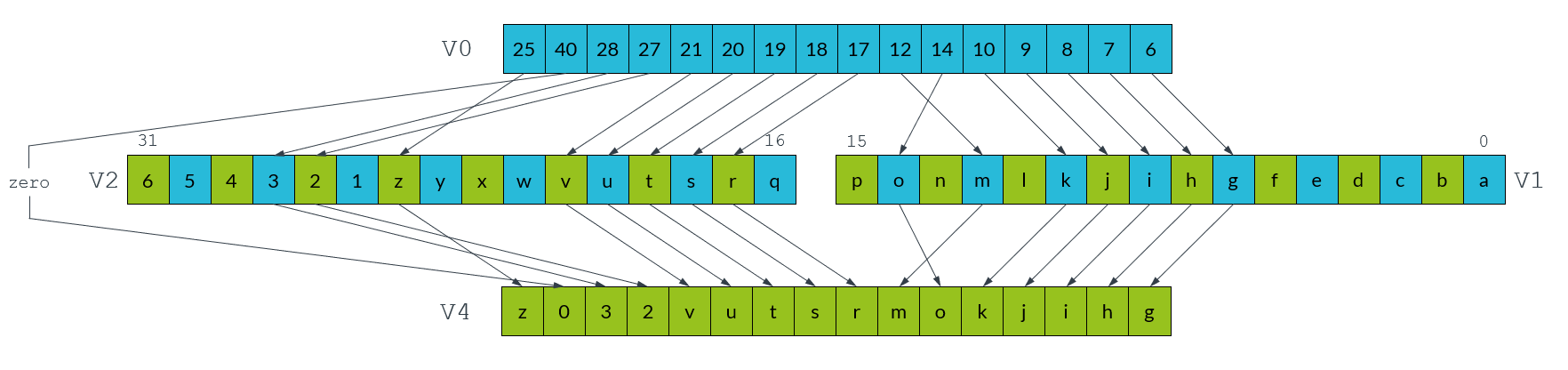The `TBL` and `TBX` instructions only differ in how they handle out of range indices. `TBL` writes a zero if an index is out-of-range, while `TBX` leaves the original value unchanged in the destination register. In the above example, lane 14 in V0 contains the lookup value, 40. Because the lookup table only contains two registers, the range of indices is 0-31. Lane 14 in the destination vector is therefore set to zero.

The `TBL` and `TBX` instructions are very powerful, so only use these instructions when necessary. On most systems a short sequence of fixed pattern permutations is faster.